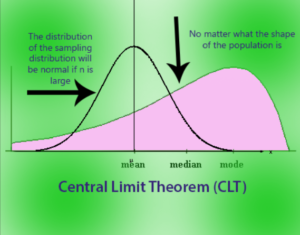# Definitions

Empirical Rule

According to empirical rule, for a normal distribution, almost all data will fall within three standard deviations of the mean. Thus, the empirical rule shows that 68% will fall within the first standard deviation, 95% within the first two standard deviations, and 99.7% will fall within the first three standard deviations of the distribution’s average.

Central Limit Theorem

Central limit theorem (CLT) is a statistical theory that states that given a sufficiently large sample size from a population with a finite level of variance, the mean of all samples from the same population will be approximately equal to the mean of the population. Furthermore, all of the samples will follow an approximate normal distribution pattern, with all variances being approximately equal to the variance of the population divided by each sample’s size.Degree Of Freedom

DOF is the total no of valthat are free to take decision, in most of the cases the DOF is no of observation -1.

F-Value

An f value (also known as an f statistic) is a random variable that has an F distribution.

Here are the steps required to compute an f value:

• Select a random sample of size n1 from a normal population, having a standard deviation equal to σ1.
• Select an independent random sample of size n2 from a normal population, having a standard deviation equal to σ2.
• The f value is the ratio of s1212 and s2222. Thus, f = [ s1212 ] / [ s2222]